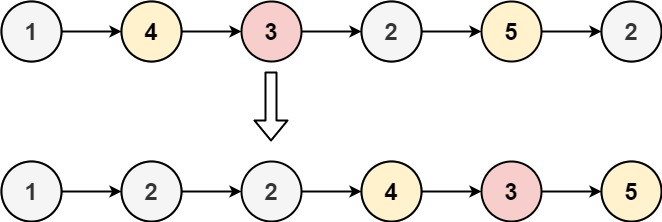86. Partition List

Given the `head` of a linked list and a value `x`, partition it such that all nodes less than `x` come before nodes greater than or equal to `x`.

You should preserve the original relative order of the nodes in each of the two partitions.

Example 1:```Input: head = [1,4,3,2,5,2], x = 3
Output: [1,2,2,4,3,5]
```

Example 2:

```Input: head = [2,1], x = 2
Output: [1,2]
```

Constraints:

• The number of nodes in the tree is in the range `[0, 200]`.
• `-100 <= Node.val <= 100`
• `-200 <= x <= 200`

86. Partition List
``````struct Solution;
use rustgym_util::*;

impl Solution {
let mut left: Vec<i32> = vec![];
let mut right: Vec<i32> = vec![];
while let Some(node) = head {
let val = node.val;
if val < x {
left.push(val);
} else {
right.push(val);
}
}
let mut res = None;
while let Some(val) = right.pop() {
}
while let Some(val) = left.pop() {